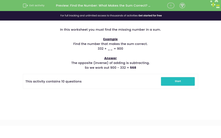# Use the Inverse Operation to Find the Answer

In this worksheet, students will find the missing number in a calculation using subtraction.Key stage:  KS 2

Curriculum topic:   Number: Addition and Subtraction

Curriculum subtopic:   Use Correct Methods to Problem Solve

Difficulty level:#### Worksheet Overview

In this activity, we will be finding the missing number in a calculation.

Example

Find the number that makes the calculation correct.

332 + __ = 900

The opposite (inverse) of adding is subtracting.

So we work out 900 - 332 = 568

Here is a simpler example:

400 + ___ = 600

The inverse operation is:

600 - 400 = 200

Does that make sense?Let's practise this skill in the following questions.

### What is EdPlace?

We're your National Curriculum aligned online education content provider helping each child succeed in English, maths and science from year 1 to GCSE. With an EdPlace account you’ll be able to track and measure progress, helping each child achieve their best. We build confidence and attainment by personalising each child’s learning at a level that suits them.

Get started••••